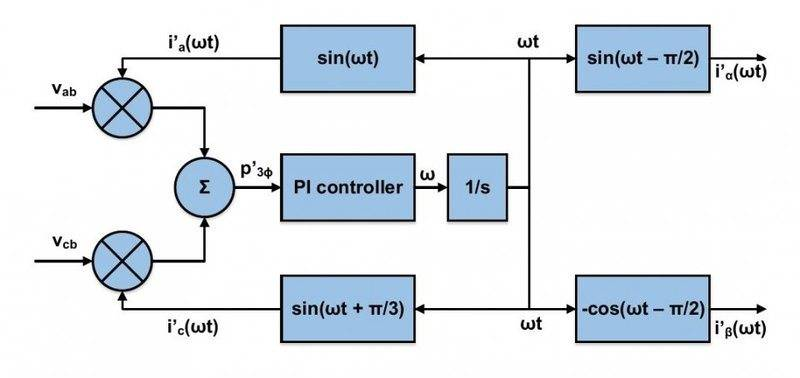# Phase Locked Loop

I have some trouble fully understanding the PLL block diagram shown below in the figure. The PLL circuit is used to generate currents (i alpha and i beta) which are in phase with the positive fundamental sequence of the voltage.

I am using the book "Instantaneous Power Theory and Applications to Power Condition" which I found as a pdf on the web.

The authors write:
• The only way for the PLL to reach a stable point is if the input to the PI controller in steady state has an average value of zero.
For the average power to be zero in steady state the current ia and voltage va has to be orthogonal to each other, i.e. $cos(\phi) = 0$.

The author also write that the frequency $\omega$ has to be equal to the system frequency and the current and voltages has to be orthogonal to reach a stable point of operation.

Why must $\omega$ be equal to system frequency to reach a stable point of operation?

I would appreciate if someone help me fully understood how this circuit works

EDIT: I forgot to write 2pi/3 at the left bottom block, i wrote pi/3.jim hardy
Gold Member
Dearly Missed
the best book i know of for PLL's is Signetics from 1972.

it's archived at https://archive.org/details/bitsavers_signeticsdcsPLLApplications_5800304

i saved a copy in my 'electronics' folder. Signetics NE565 datasheet is a companion piece.

Why must ω\omega be equal to system frequency to reach a stable point of operation?

That's what PLL's do, lock on to a frequency. Being out of sync is like grinding gear teeth. Read about capture transient in that Signetics book.

•OliskaP
jim hardy
•